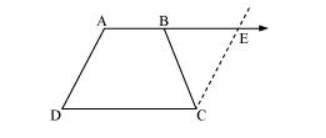# ABCD is a trapezium in which AB || CD and AD = BC

Question. ABCD is a trapezium in which AB || CD and AD = BC (see the given figure). Show that(i) $\angle \mathrm{A}=\angle \mathrm{B}$

(ii) $\angle \mathrm{C}=\angle \mathrm{D}$

(iii) $\triangle \mathrm{ABC} \cong \triangle \mathrm{BAD}$

(iv) diagonal $A C=$ diagonal $B D$

Solution:

Let us extend AB. Then, draw a line through C, which is parallel to AD, intersecting AE at point E. It is clear that AECD is a parallelogram.

(i) $A D=C E$ (Opposite sides of parallelogram $A E C D$ )

However, $A D=B C$ (Given)

Therefore, $B C=C E$

$\angle C E B=\angle C B E$ (Angle opposite to equal sides are also equal)

Consider parallel lines $A D$ and $C E . A E$ is the transversal line for them.

$\angle A+\angle C E B=180^{\circ}$ (Angles on the same side of transversal)

$\angle \mathrm{A}+\angle \mathrm{CBE}=180^{\circ}($ Using the relation $\angle \mathrm{CEB}=\angle \mathrm{CBE}) \ldots(1)$

However, $\angle B+\angle C B E=180^{\circ}$ (Linear pair angles) ... (2)

From equations (1) and (2), we obtain

$\angle \mathrm{A}=\angle \mathrm{B}$

(ii) $\mathrm{AB} \| \mathrm{CD}$

$\angle \mathrm{A}+\angle \mathrm{D}=180^{\circ}$ (Angles on the same side of the transversal)

Also, $\angle C+\angle B=180^{\circ}$ (Angles on the same side of the transversal)

$\therefore \angle \mathrm{A}+\angle \mathrm{D}=\angle \mathrm{C}+\angle \mathrm{B}$

However, $\angle \mathrm{A}=\angle \mathrm{B}$ [Using the result obtained in (i)]

$\therefore \angle \mathrm{C}=\angle D$

(iii) In $\triangle \mathrm{ABC}$ and $\triangle \mathrm{BAD}$,

$A B=B A$ (Common side)

$\mathrm{BC}=\mathrm{AD}$ (Given)

$\angle \mathrm{B}=\angle \mathrm{A}$ (Proved before)

$\therefore \triangle \mathrm{ABC} \cong \triangle \mathrm{BAD}(\mathrm{SAS}$ congruence rule $)$

$\triangle \mathrm{ABC} \cong \triangle \mathrm{BAD}$
$\therefore \mathrm{AC}=\mathrm{BD}(\mathrm{By} \mathrm{CPCT})$